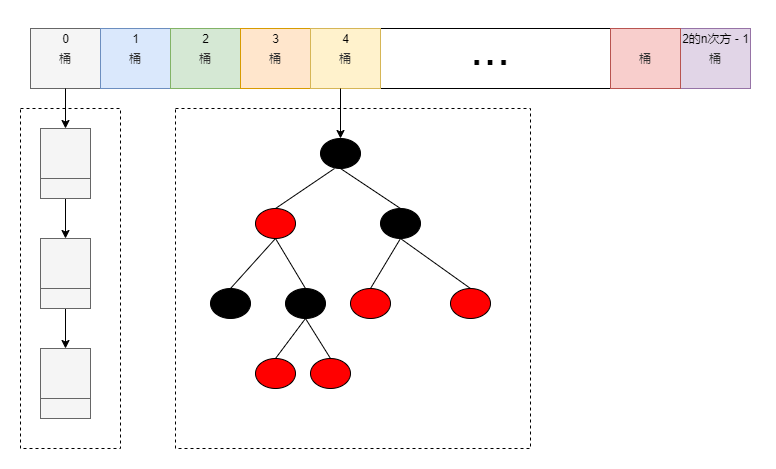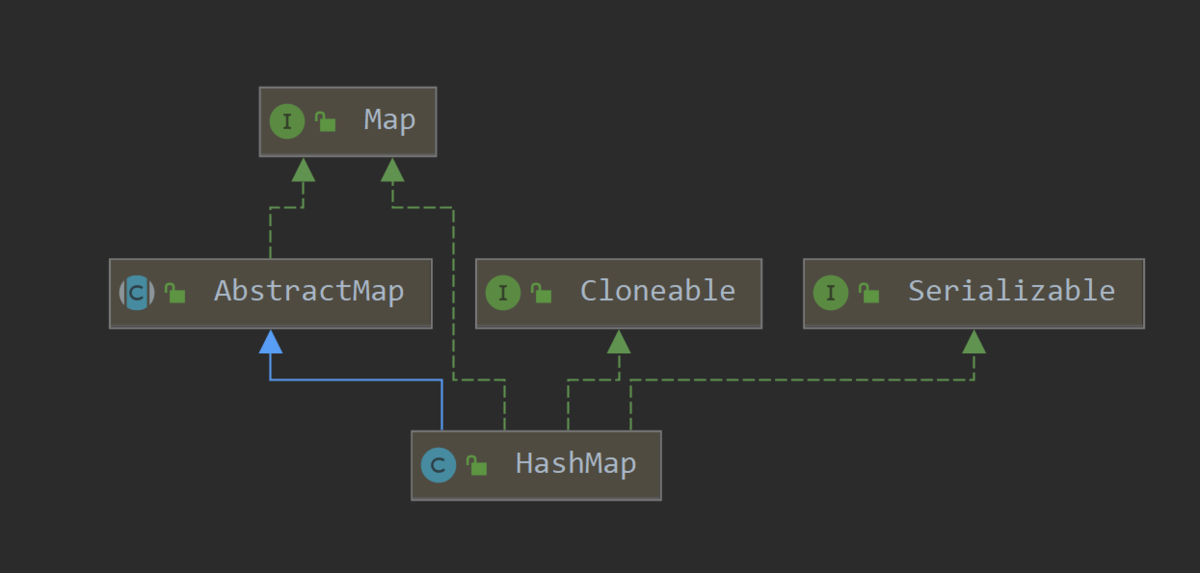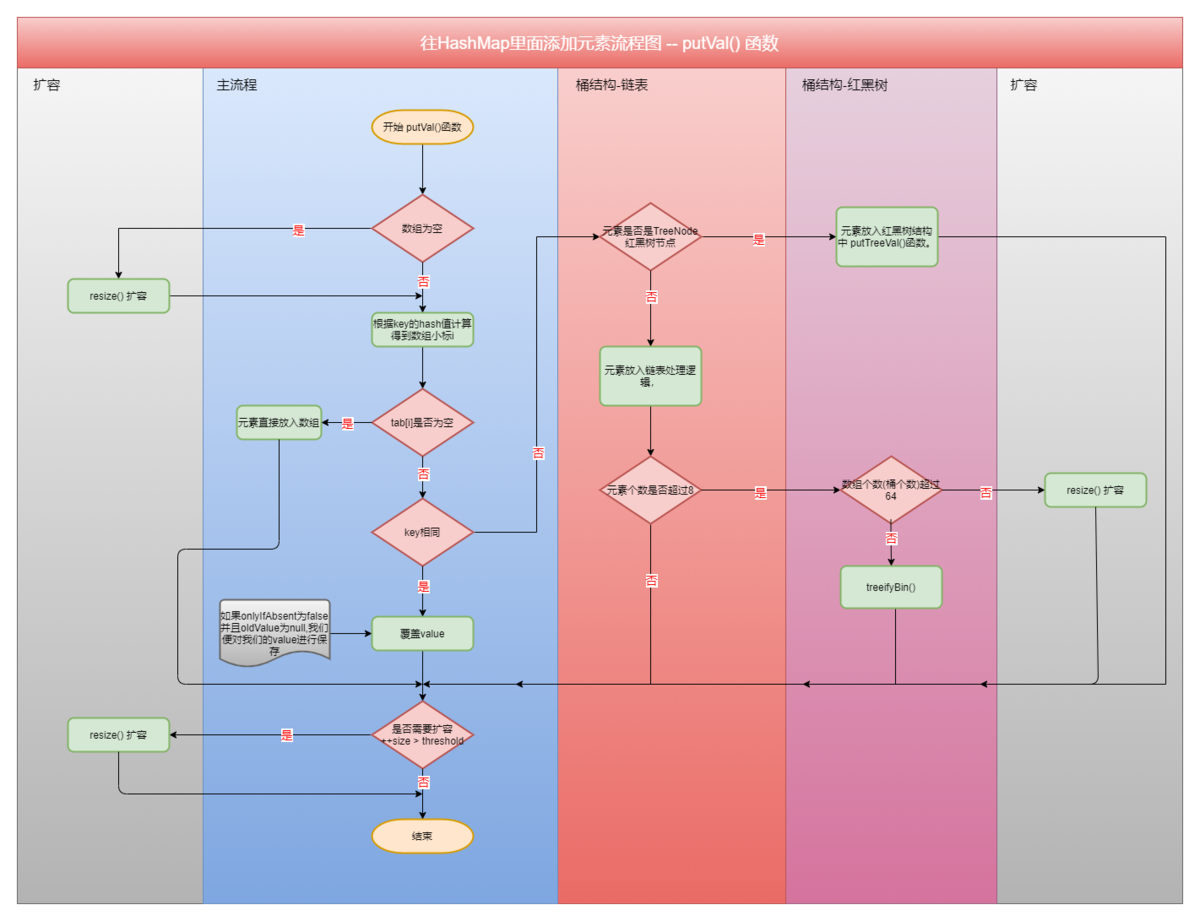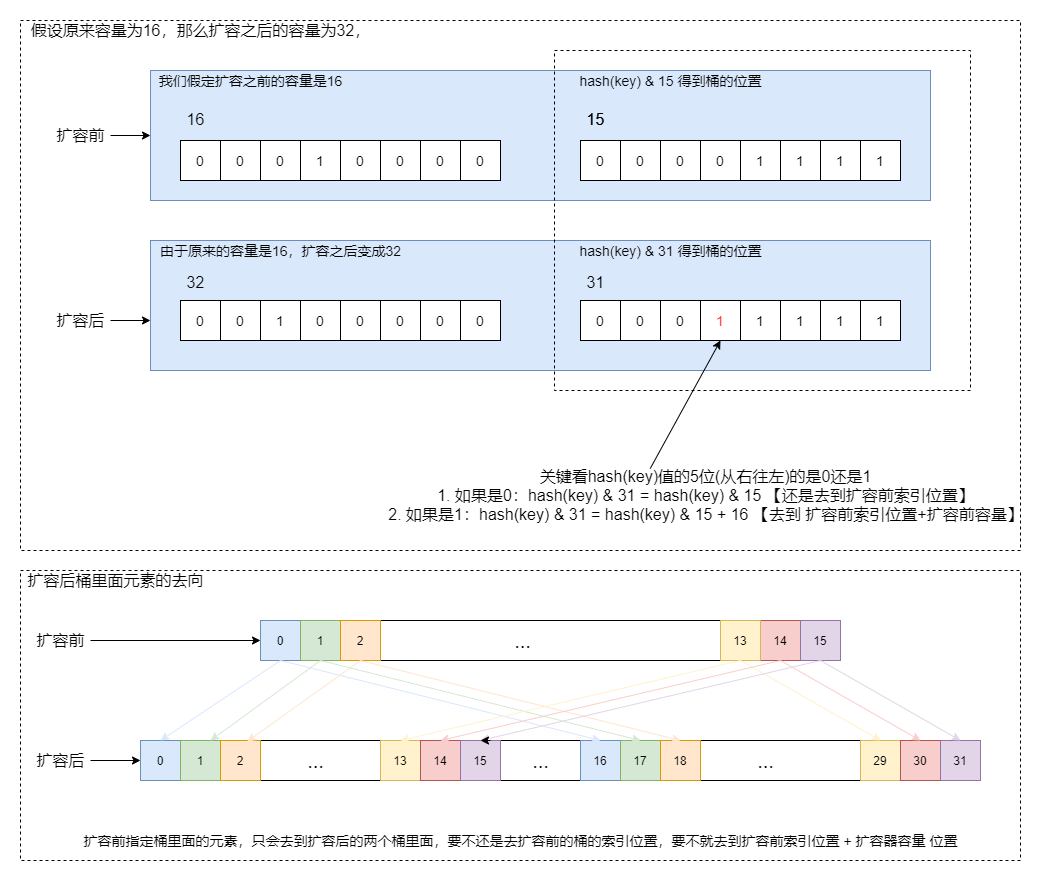# Java- 技术专题 -JDK8-HashMap 的实现原理# 一 HashMap底层存储结构HashMap底层结构采用（数组）+（链表 or 红黑树）的形式来存储节点。

• 首先HashMap是一个数组，而且数组里面每个位置可以放入多个元素，形象一点，咱们把数组的这些个位置称为桶。HashMap里面每个元素通过key值取hash在 & （数组长度容量-1）就可以唯一确定该元素属于哪个桶。

• HashMap为了最大限度的提高效率，桶可能是链表也可能是红黑树。开始桶里面元素不多的时候采用 链表形式保存。后续随着HashMap里面的元素越来越多，桶里面里面的元素元素也越来越多，当元素 个数>8（TREEIFY_THRESHOLD）并且数组的长度>64（MIN_TREEIFY_CAPACITY）的时候，会把 链表变成红黑树（树化）；

• 如果桶当前采用的是红黑树保存节点元素信息随着节点个数的减少（HashMap remove操作），当节

HashMap的桶有了链表为啥还要转换为红黑树？HashMap源码大神考虑到链表太长的话。节点元素的查找效率不高。所以有链表转红黑树，红黑树转链表的操作。可能你又会想为啥不用平衡二叉树来替换红黑树。那是因为HashMap源码大神兼顾了节点的插入删除效率和节点的查询效率。

# 二 HashMap源码分析

了解了HashMap的存储结构之后，咱们着重对HashMap添加节点的过程做一个简单的分析。我相信只要咱们搞懂了HashMap里面添加元素的过程。HashMap里面大部分的实现逻辑咱们都能搞懂。## 2.1 准备工作

• HashMap里面一些关键属性介绍

/*** 默认初始容量(默认数组桶的个数)16-必须为2的幂。*/static final int DEFAULT_INITIAL_CAPACITY = 1 << 4; // aka 16﻿/*** 最大容量2的30次方。桶的最大个数*/static final int MAXIMUM_CAPACITY = 1 << 30;﻿/*** 构造函数中未指定时使用的负载系数，*/static final float DEFAULT_LOAD_FACTOR = 0.75f;﻿/*** 桶结构树化需要两个条件* 1. 桶里面元素个数大于TREEIFY_THRESHOLD* 2. 桶的个数(数组的长度)大于MIN_TREEIFY_CAPACITY*/static final int TREEIFY_THRESHOLD = 8;﻿/*** 如果当前桶采用的是红黑树保存节点，当桶里面的元素小于该值时，红黑树降级为链表。*/static final int UNTREEIFY_THRESHOLD = 6;﻿/*** 桶结构树化需要两个条件* 1. 桶里面元素个数大于TREEIFY_THRESHOLD* 2. 桶的个数(数组的长度)大于MIN_TREEIFY_CAPACITY*/static final int MIN_TREEIFY_CAPACITY = 64;﻿/*** HashMap数组。长度必须是2的幂次方*/transient Node<K,V>[] table;﻿/*** HashMap中所有元素节点的个数*/transient int size;﻿/*** 扩容（重hash）或者map结构修改的次数*/transient int modCount;﻿/*** 扩容阈值【当HashMap的所有元素个数大于threashold时会进行扩容操作】，threshold=容量*loadFactor(装载因子)*/int threshold;﻿/*** 装载因子，用来衡量HashMap满的程度。默认为0.75f*/final float loadFactor;
• HashMap容量(数组大小，桶的个数)永远是2的整数次幂

时时刻刻要记住HashMap的容量(桶的个数)永远是2的整数次幂。初始容量16，每次扩容之后的容量都是前一次容量的两倍。比如当前容量是16扩容一次编程32，再扩容一次变成64。

而且如果我们在new HashMap的时候，给了初始容量，但是给定的容量不是2的整数次幂，构造函数内部也会调用tableSizeFor()函数转换成2的整数次幂的。比如：传递3会转换成4，传递13会转换成16

/*** 返回大于输入参数且最近的2的整数次幂的数* 比如 cap = 2 的时候返回 2*     cap = 3 的时候返回 4*     cap = 9 的时候返回 16*/static final int tableSizeFor(int cap) {    int n = cap - 1;    n |= n >>> 1;    n |= n >>> 2;    n |= n >>> 4;    n |= n >>> 8;    n |= n >>> 16;    return (n < 0) ? 1 : (n >= MAXIMUM_CAPACITY) ? MAXIMUM_CAPACITY : n + 1;}
• HashMap里面的元素桶的位置是怎么确定的(HashMap元素在数组中的索引位置)

很简单通过对元素的key做hash处理在 &（容量-1），就精确找到找个这个元素应该放入哪个桶中。

## 2.2 resize()扩容

一定要时刻记住HashMap的容量(数组的大小，我们也说桶的个数)永远都是2的整数次幂，扩容就是把容量扩大一倍。扩容之后的容量=原来容量*2。（桶的个数翻倍）就算你new HashMap()的时候给定的容量不是2的整数次幂。HashMap内部也是会通过tableSizeFor()函数把容量转换成2的整数次幂

HashMap确定元素属于哪个桶是通过对该元素的key取hash之后再 & （容量-1）。这样就找到了桶的位置（其实是数组的下标）。

扩容前后桶的关系也要特别注意，扩容前属于同一个桶(桶的索引位置相同)里面的元素，在扩容之后只会有两个桶来放他们：要不还保留扩容前的桶的索引位置，要不就是通过扩容前的索引位置+扩容前的容量得和值确定位置。我们举个例子，假设原来的容量是16，那么扩容之后的容量就是32。有了上面的理解，接下来，我们看HashMap的扩容逻辑就简单了，我们就直接贴代码，加注释了。

/*** HashMap扩容函数* 1. 容量，扩容阙值等都相应的扩大两倍* 2. 扩容前每个桶里面的元素，重新放入扩容之后对应桶里面*/final Node<K,V>[] resize() {    Node<K,V>[] oldTab = table;    int oldCap = (oldTab == null) ? 0 : oldTab.length;// 扩容前容量(扩容前桶的个数)    int oldThr = threshold; // 扩容前阈值    int newCap, newThr = 0; // 扩容后容量，扩容后阈值    if (oldCap > 0) {        if (oldCap >= MAXIMUM_CAPACITY){            //如果扩容前容量大于最大容量            threshold = Integer.MAX_VALUE;             // 阈值设置为最大值            return oldTab; // 直接返回不需要扩容        }        // 扩容，容量，阈值都扩大一倍        else if ((newCap = oldCap << 1) < MAXIMUM_CAPACITY &&         			oldCap >= DEFAULT_INITIAL_CAPACITY)            newThr = oldThr << 1; // double threshold    }    else if (oldThr > 0) // initial capacity was placed in threshold        // 初始容量设置为阈值        newCap = oldThr;    else {                       // 零初始阈值表示使用默认值        newCap = DEFAULT_INITIAL_CAPACITY;        newThr = (int)(DEFAULT_LOAD_FACTOR * DEFAULT_INITIAL_CAPACITY);    }    if (newThr == 0) {        // 如果扩容后的阈值等于0，重新计算扩容后的阈值 = 扩容后的容量*默认扩容因子        float ft = (float)newCap * loadFactor;        newThr = (newCap < MAXIMUM_CAPACITY&&ft<(float)MAXIMUM_CAPACITY?                 (int)ft : Integer.MAX_VALUE);    }    threshold = newThr; //扩容后的阈值赋值给阈值    @SuppressWarnings({"rawtypes","unchecked"})    // 创建扩容之后的桶数组    Node<K,V>[] newTab = (Node<K,V>[])new Node[newCap];     table = newTab; // 赋值给table    if (oldTab != null) {        // 遍历扩容之前的桶数组，遍历扩容前的每个桶        for (int j = 0; j < oldCap; ++j){            Node<K,V> e;            // 如果扩容之前的桶里面有元素            if ((e = oldTab[j]) != null){                oldTab[j] = null;                if (e.next == null)                // 扩容前桶里面只有一个元素，直接放到新数组中(是可以直接放的，                // 因为不会有别的桶的元素放到这个位置的)                    newTab[e.hash & (newCap - 1)] = e;                else if (e instanceof TreeNode)                    // 扩容前桶是红黑树，做对应红黑树的拆分处理                    ((TreeNode<K,V>)e).split(this, newTab, j, oldCap);                else {                     // preserve order                    // 扩容前桶是链表，这里要再次强调下，扩容前同一个桶里面的元素，扩容之后只会往两个桶放这些元素，我们前面讲的很清楚。要不就是还是保留原来的索引位置，要不就是原来的索引位置在加上扩容前的容量                    Node<K,V> loHead = null, loTail = null;                     // 保留扩容前索引位置的，头和尾                    Node<K,V> hiHead = null, hiTail = null;                     // 扩容索引位置+扩容前容量的位置，头和尾                    Node<K,V> next;                    do {                        next = e.next;                        if ((e.hash & oldCap) == 0) { // 扩容后桶的索引位置还是扩容前索引位置                            if (loTail == null)                                loHead = e;                            else                                loTail.next = e;                            loTail = e;                        }                        else {                         // 扩容后桶的索引位置 = 扩容前索引位置 + 扩容前容量                            if (hiTail == null)                                hiHead = e;                            else                                hiTail.next = e;                            hiTail = e;                        }                    } while ((e = next) != null);                    if (loTail != null) {                        loTail.next = null;                        newTab[j] = loHead;// 把链表的头告诉桶                    }                    if (hiTail != null) {                        hiTail.next = null;                        newTab[j + oldCap] = hiHead;// 把链表的头告诉桶                    }                }            }        }    }    return newTab;}

﻿

/*** 红黑树的拆分处理*/final void split(HashMap<K,V> map, Node<K,V>[] tab, int index, int bit) {    TreeNode<K,V> b = this;    // Relink into lo and hi lists, preserving order    /*    	这里需要再次强调一点，在扩容的过程中，桶一个桶里面的元素，在扩容之后只会在两个位置，要么还是保留扩容前桶索引位置，要么去到扩容前桶索引位置+扩容前容量    	什么意思，我们举个例子。假设原来的HashMap的数组长度是16，那么扩容之后的数组的长度是32    	在比如这个时候，扩容前HashMap，数组下标3里面的所有的元素。在扩容之后。要么在扩容之后数组下标3里面要么在下标 3+16=19里面    */    TreeNode<K,V> loHead = null, loTail = null; // 低位(还是放入扩容器前桶的索引位置)，loHead首节点，loTail尾节点    TreeNode<K,V> hiHead = null, hiTail = null; // 高位(放入扩容前桶索引位置+扩容前容量)，hiHead首节点，hiTail尾节点    int lc = 0, hc = 0;    /*    先把红黑树里面的每个元素，找到对应的数组的数组下标，并且组成一个双向链表    */    for (TreeNode<K,V> e = b, next; e != null; e = next) {        next = (TreeNode<K,V>)e.next;        e.next = null;        if ((e.hash & bit) == 0) { // 扩容之后还是保留原来的索引位置            if ((e.prev = loTail) == null)                loHead = e;            else                loTail.next = e;            loTail = e;            ++lc;        }        else { // 扩容之后去到 扩容前索引位置+扩容前容量            if ((e.prev = hiTail) == null)                hiHead = e;            else                hiTail.next = e;            hiTail = e;            ++hc;        }    }﻿    if (loHead != null) {        if (lc <= UNTREEIFY_THRESHOLD)            // 如果loHead上的树节点小于等于6个那就去树化变回链表            tab[index] = loHead.untreeify(map);        else {            tab[index] = loHead;            if (hiHead != null) // (else is already treeified)                // 转换成红黑树                loHead.treeify(tab);        }    }    if (hiHead != null) {        if (hc <= UNTREEIFY_THRESHOLD)            // 如果loHead上的树节点小于等于6个那就去树化变回链表            tab[index + bit] = hiHead.untreeify(map);        else {            tab[index + bit] = hiHead;            if (loHead != null)                // 转换成红黑树                hiHead.treeify(tab);        }    }}

## 2.3 treeifyBin()树化

HashMap的树化，就是把链表转换为红黑树。当往HashMap里面添加元素的时候，随着桶里面元素的增加，当桶里面元素的个数大于8（TREEIFY_THRESHOLD），并且HashMap的容量大于64（MIN_TREEIFY_CAPACITY）的时候才会把链表树化成红黑树。

### 红黑树特性

• 左子节点小于右子节点。（方便搜索）

• 节点是红色或者黑色。

• 根节点是黑色。

• 每个叶子的节点都是黑色的空节点（NULL）。

• 每个红色节点的两个子节点都是黑色。（从每个叶子节点到根节点的所有路径上不能有两个连续的红色节点）

• 从任一节点到其每个叶子节点的所有路径都包含相同数目的黑色节点。

/*** treeifyBin方法用于把桶的两遍转换为红黑树*/final void treeifyBin(Node<K,V>[] tab, int hash) {    int n, index; Node<K,V> e;    if (tab == null || (n = tab.length) < MIN_TREEIFY_CAPACITY)        // 虽然桶里面的元素大于8了，但是容量还没到到64（MIN_TREEIFY_CAPACITY），还是进行扩容        resize();    else if ((e = tab[index = (n - 1) & hash]) != null) {        TreeNode<K,V> hd = null, tl = null; // hd首节点，tl尾节点        do {            // Entry做结构转换每个节点都转换成TreeNode，先组成一个双向链表(每个节点都有prev,next)            TreeNode<K,V> p = replacementTreeNode(e, null);            if (tl == null)                hd = p;            else {                p.prev = tl;                tl.next = p;            }            tl = p;        } while ((e = e.next) != null);        // 上面部分组成了一个双向链表(每个节点都有prev,next)        // 把组成的双向链表，红黑树树化，转换成一个红黑树        if ((tab[index] = hd) != null)            hd.treeify(tab);    }}

﻿

/*** 红黑树的树化过程*/final void treeify(Node<K,V>[] tab) {    TreeNode<K,V> root = null; // 红黑树的根节点    /*    依次遍历，双向链表的每个节点。root是组成之后红黑树的根节点，    x是当前想放入红黑树的节点，next是下一个想放入红黑树的节点    */    for (TreeNode<K,V> x = this, next; x != null; x = next) {        next = (TreeNode<K,V>)x.next;        x.left = x.right = null; // 当前操作节点的左节点，右节点清空        if (root == null) {            // 处理第一个节点            x.parent = null;            x.red = false;            root = x;        }        else {            K k = x.key; // 准备放入红黑树节点对应的key            int h = x.hash; // 准备放入红黑树节点对应的hash            Class<?> kc = null;            for (TreeNode<K,V> p = root;;) { // 从红黑树的根节点开始遍历，p是红黑树中当前遍历到的节点                int dir, ph; // dir:-1或0 左孩子，1 右孩子(红黑树也是二叉树，要保证左孩子小于右孩子)                K pk = p.key;                /*                比较hash的大小，红黑树也是二叉树，要保证左孩子小于右孩子                */                if ((ph = p.hash) > h) // 红黑树遍历到的节点的hash大于当前准备放入节点的hash。所以需要放入的节点肯定放在红黑树遍历到的当前节点的左孩子里面                    dir = -1;                else if (ph < h) // 红黑树遍历到的节点的hash小于当前准备放入节点的hash。所以需要放入的节点肯定放在红黑树遍历到的当前节点的右孩子里面                    dir = 1;                else if ((kc == null &&                          (kc = comparableClassFor(k)) == null) ||                         (dir = compareComparables(kc, k, pk)) == 0) // 相等的情况下，左其他方式的比较                    dir = tieBreakOrder(k, pk);﻿                TreeNode<K,V> xp = p;                /*                找到需要放入红黑树节点的位置，                */                if ((p = (dir <= 0) ? p.left : p.right) == null) {                    x.parent = xp;                    if (dir <= 0)                        xp.left = x; // 左孩子                    else                        xp.right = x; // 右孩子                    root = balanceInsertion(root, x); // 红黑树平衡操作-其实上面一大段还只是形成了二叉树，只有对二叉树做了红黑树的平衡操作，才能成为红黑树                    break;                }            }        }    }    moveRootToFront(tab, root);}

﻿

/**红黑树的平衡算法，当树结构中新插入了一个节点后，要对树进行重新的结构化，以保证树始终维持红黑树特性*/static <K,V> TreeNode<K,V> balanceInsertion(TreeNode<K,V> root,                                            TreeNode<K,V> x) {    x.red = true; // 新插入的节点标记为红色节点    /*    这一步即定义了变量，又开启了循环，循环没有控制条件，只能从内部跳出    xp：父节点、xpp：爷爷节点、xppl：左叔叔节点、xppr：右叔叔节点    */    for (TreeNode<K,V> xp, xpp, xppl, xppr;;) {        // 如果父节点为空、说明当前节点就是根节点，那么把当前节点标为黑色，返回当前节点        if ((xp = x.parent) == null) {            x.red = false;            return x;        }        else if (!xp.red || (xpp = xp.parent) == null)            return root;        if (xp == (xppl = xpp.left)) { // 父节点是爷爷节点的左孩子            if ((xppr = xpp.right) != null && xppr.red) { // 如果右叔叔不为空 并且 为红色                xppr.red = false; // 右叔叔置为黑色                xp.red = false; // 父节点置为黑色                xpp.red = true; // 爷爷节点置为红色                x = xpp;            }            else { // 如果右叔叔为空 或者 为黑色                if (x == xp.right) { // 如果当前节点是父节点的右孩子                    root = rotateLeft(root, x = xp); // 父节点左旋                    xpp = (xp = x.parent) == null ? null : xp.parent; // 获取爷爷节点                }                if (xp != null) { // 如果父节点不为空                    xp.red = false; // 父节点 置为黑色                    if (xpp != null) { // 爷爷节点不为空                        xpp.red = true; // 爷爷节点置为 红色                        root = rotateRight(root, xpp); //爷爷节点右旋                    }                }            }        }        else { // 父节点是爷爷节点的右孩子            if (xppl != null && xppl.red) { // 如果左叔叔是红色                xppl.red = false; // 左叔叔置为 黑色                xp.red = false; // 父节点置为黑色                xpp.red = true; // 爷爷置为红色                x = xpp;            }            else { // 如果左叔叔为空或者是黑色                if (x == xp.left) { // 如果当前节点是个左孩子                    root = rotateRight(root, x = xp); // 针对父节点做右旋                    xpp = (xp = x.parent) == null ? null : xp.parent; // 获取爷爷节点                }                if (xp != null) { // 如果父节点不为空                    xp.red = false; // 父节点置为黑色                    if (xpp != null) { //如果爷爷节点不为空                        xpp.red = true; // 爷爷节点置为红色                        root = rotateLeft(root, xpp); // 针对爷爷节点做左旋                    }                }            }        }    }}

# 三 总结

通过对HashMap的实现做简单的分析，咱们可以总结出如下信息：

• HashMap的初始容量是16。

• HashMap的容量永远都是2的整数次幂，扩容之后的容量 = 扩容之前的容量*2。

• HashMap扩容时机，当HashMap里面的元素个数 > 容量 * loadFactor (默认0.75)。

• HashMap树化时机(链表转红黑树)，当桶里面的元素个数 >= 8(TREEIFY_THRESHOLD)，并且HashMap的容量 > 64(MIN_TREEIFY_CAPACITY)。

• HashMap去树化的时机(红黑树转链表)，当红黑树里面的元素个数 <= 6(UNTREEIFY_THRESHOLD)。

• HashMap是线程不安全的，我们在分析过程中没有看到任何线程安全的保障。

﻿## 评论